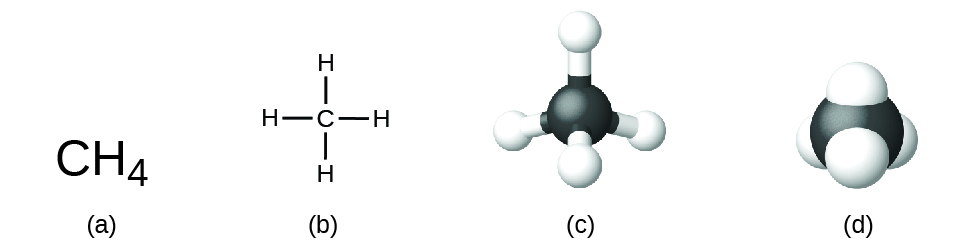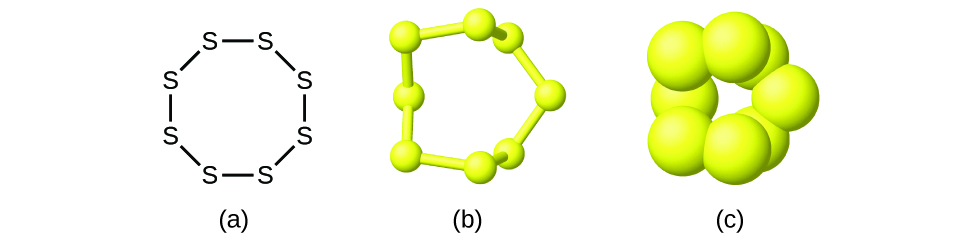# 1.9 Chemical formulas

 Page 1 / 10
By the end of this section, you will be able to:
• Symbolize the composition of molecules using molecular formulas and empirical formulas
• Represent the bonding arrangement of atoms within molecules using structural formulas

A molecular formula    is a representation of a molecule that uses chemical symbols to indicate the types of atoms followed by subscripts to show the number of atoms of each type in the molecule. (A subscript is used only when more than one atom of a given type is present.) Molecular formulas are also used as abbreviations for the names of compounds.

The structural formula    for a compound gives the same information as its molecular formula (the types and numbers of atoms in the molecule) but also shows how the atoms are connected in the molecule. The structural formula for methane contains symbols for one C atom and four H atoms, indicating the number of atoms in the molecule ( [link] ). The lines represent bonds that hold the atoms together. (A chemical bond is an attraction between atoms or ions that holds them together in a molecule or a crystal.) We will discuss chemical bonds and see how to predict the arrangement of atoms in a molecule later. For now, simply know that the lines are an indication of how the atoms are connected in a molecule. A ball-and-stick model shows the geometric arrangement of the atoms with atomic sizes not to scale, and a space-filling model shows the relative sizes of the atoms.A methane molecule can be represented as (a) a molecular formula, (b) a structural formula, (c) a ball-and-stick model, and (d) a space-filling model. Carbon and hydrogen atoms are represented by black and white spheres, respectively.

Although many elements consist of discrete, individual atoms, some exist as molecules made up of two or more atoms of the element chemically bonded together. For example, most samples of the elements hydrogen, oxygen, and nitrogen are composed of molecules that contain two atoms each (called diatomic molecules) and thus have the molecular formulas H 2 , O 2 , and N 2 , respectively. Other elements commonly found as diatomic molecules are fluorine (F 2 ), chlorine (Cl 2 ), bromine (Br 2 ), and iodine (I 2 ). The most common form of the element sulfur is composed of molecules that consist of eight atoms of sulfur; its molecular formula is S 8 ( [link] ).A molecule of sulfur is composed of eight sulfur atoms and is therefore written as S 8 . It can be represented as (a) a structural formula, (b) a ball-and-stick model, and (c) a space-filling model. Sulfur atoms are represented by yellow spheres.

It is important to note that a subscript following a symbol and a number in front of a symbol do not represent the same thing; for example, H 2 and 2H represent distinctly different species. H 2 is a molecular formula; it represents a diatomic molecule of hydrogen, consisting of two atoms of the element that are chemically bonded together. The expression 2H, on the other hand, indicates two separate hydrogen atoms that are not combined as a unit. The expression 2H 2 represents two molecules of diatomic hydrogen ( [link] ).

what is motion?
where the solving of questions of this topic?
According to Nernst's distribution law there are about two solvents in which solutes undergo equilibria. But i don't understand how can you know which of two solvents goes bottom and one top? I real want to understand b'coz some books do say why they prefer one to top/bottom.
I need chapter 25 last topic
What is physics?
Abdulaziz
physics is the study of matter and energy in space and time and how they related to each other
Manzoor
interaction of matter and eneegy....
Abdullah
thanks for correcting me bro
Manzoor
What is electrostatics bassically?
study of charge at rest
wamis
A branch in physics that deals with statics electricity
Akona
what is PN junction?
Manzoor
please I don't understand the solution of the first example as in d working
what's the question? Write it here.
SABYASACHI
a cold body of 100°C and a hot body is of 100°F . Transfer heat = ?
you are given two metal spheres mounted on portable insulating support. Find a way to give them equal and opposite charges. you may use a glass rod rubbed with silk but may not touch it to the spheres. Do the spheres have to be of equal size for your method to work?
what is emotion?
Abdulaziz
in the 2nd example, for chapter 8.2 on page 3/3, I don't understand where the value 48uC comes from, I just couldn't get that value in my calculator.
are you talking about the capacitance combination problem
sam
please write the problem or send a snap of th page....I don't have the book in my vicinity.
SABYASACHI
yes, the 2nd example called Network of Capacitors on page 3/3 of section 8.2.
Anita
12 V = (Q1/12uF)+(Q1/6uF). So, Q1 = 12x4 = 48 uC.
sam
ohhhh OK thanks so much!!!!!!!
Anita
hello guys,, I'm asking to know something about, How can i know which solvent goes down and which does up in determination of partion coefficient(Nernst's distribution law). Please Need help because i have seen many contradictions via few of text books even some videos on youtube they don't say
Elia
what is electromagnetic force. do electric and magnetic force happen differently
yes
yes
Pranay
why
Godson
how?
Godson
when electric charge exert force on another electric charge then this force is known as electrostatic force and when a magnet exert force on another magnet then this force is known as magnetic force and when force exerted on magnet due to varying electric field then this electromagnetic force
Ilyas
Yes
Akona
derived the electric potential due to disk of charge
how can we derived potential electric due to the disk
aron
how can you derived electric potential of a disk
aron
how can you derived electric potential due to disk
aron
where is response?
aron
what is difference between heat and temperature?
temperature is the measure of degree of hotness or coldness. on the other hand, heat is the form of energy, which causes temperature. So we can safely say, heat is the reason and temperature is its consequence.
SABYASACHI
Heat is the reason and temperature is the consequences
Angela
how many liquid metals do we have
do we have gasses as metals
Jeffery
who knows should please tell us
yes...gallium & cesium
Idris
Hg is liquid. No metal gasses at standard temp and pressure
Shane
I don't ever understand any of this formulae
which formula
How to determine a temperature scale
what is the formula for absolute error
Nyro
how do you find theWhat are the wavelengths and energies per photon of two lines
The eyes of some reptiles are sensitive to 850 nm light. If the minimum energy to trigger the receptor at this wavelength is 3.15 x 10-14 J, what is the minimum number of 850 nm photons that must hit the receptor in order for it to be triggered?
A teaspoon of the carbohydrate sucrose contains 16 calories, what is the mass of one teaspoo of sucrose if the average number of calories for carbohydrate is 4.1 calories/g?
4. On the basis of dipole moments and/or hydrogen bonding, explain in a qualitative way the differences in the boiling points of acetone (56.2 °C) and 1-propanol (97.4 °C), which have similar molar masses
Calculate the bond order for an ion with this configuration: (?2s)2(??2s)2(?2px)2(?2py,?2pz)4(??2py,??2pz)3
Which of the following will increase the percent of HF that is converted to the fluoride ion in water? (a) addition of NaOH (b) addition of HCl (c) addition of NaFBy Abishek DevarajByByByByBy Rhodes